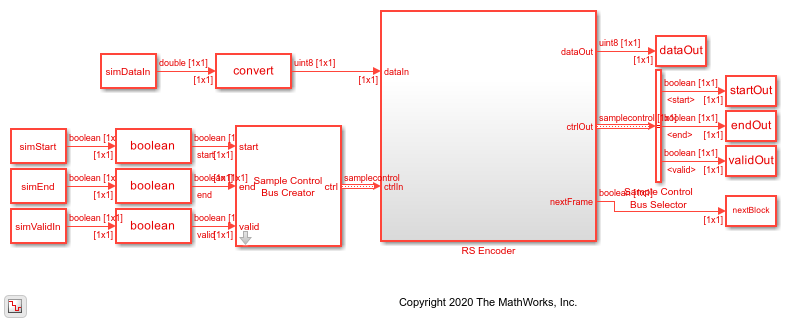# Encode message to RS codeword

This example shows how to use the RS Encoder block to encode a message to a Reed-Solomon (RS) codeword. In this example, a set of random inputs frames are generated and provided to the `comm.RSEncoder` System object. Using the `whdlFramesToSamples` function, these frames are converted into samples and provided as input to the RS Encoder block. The output of the RS Encoder block is then compared with the output of the `comm.RSEncoder` System object to check whether the encoded output codeword for the given input message is same. By default, the puncturing option is disabled in this example. To enable puncturing, set the puncturing value to `true`. This example model supports HDL code generation for the RS Encoder subsystem.

### Set Up Input Data Parameters

Set up these workspace variable for the models to use. These variables configure the RS Encoder block inside the model.

```nMessages = 3; n = 255; % Specify codeword length k = 239; % Specify message length m = n-k; % Parity length inDataType = fixdt(0,ceil(log2(n)),0); puncturing = false; % true for puncturing puncturePattern = randsrc(m,1,[0 1]); % Considered, when punturing is true shortMsg = false; % true for shortened message k1 = k-1; % Considered when shortMsg is true ```

### Generate Random Input Samples

Generate random samples using `n`, `k`, and `m` variables and provide those generated samples as input to the `comm.RSEncoder` System object.

```hRSEnc = comm.RSEncoder; hRSEnc.CodewordLength = n; hRSEnc.MessageLength = k; if isequal(shortMsg,true) hRSEnc.ShortMessageLength = k1; else k1 = k; end if isequal(puncturing,true) hRSEnc.PuncturePatternSource = "Property"; hRSEnc.PuncturePattern = puncturePattern; puncLen = n-k-sum(hRSEnc.PuncturePattern); else puncLen = 0; end data = cell(1,nMessages); refData = (zeros(k1+m-puncLen,nMessages)); for ii = 1:nMessages data{ii} = randi([0 n],k1,1); refData(:,ii) = hRSEnc(data{ii}); end refOutput = refData(:); ```

### Generate Input Control Samples for the Simulink® Model

```gapBetweenFrames = n-k; gapBetweenSamples = 0; [simDataIn, ctrlIn] = whdlFramesToSamples(data,gapBetweenSamples,gapBetweenFrames); simStart = ctrlIn(:,1); simEnd = ctrlIn(:,2); simValidIn = ctrlIn(:,3); stopTime = length(simValidIn); ```

Run the Simulink model. The block imports the workspace variables and generates the output.

```modelname = 'HDLRSEncoder'; open_system(modelname); if isequal(puncturing,true) set_param([modelname '/RS Encoder/RS Encoder'],'PuncturePatternSource','on'); set_param([modelname '/RS Encoder/RS Encoder'],'PuncturePattern',['[' num2str(puncturePattern') ']']); end out = sim(modelname); ```### Export the Simulink Block Output to the MATLAB® Workspace

The encoded samples from the RS Encoder block are exported to the MATLAB workspace.

```simOutput = dataOut(validOut); ```

### Compare the Simulink Block Output with the MATLAB Function Output

Capture the output of the RS Encoder block. Compare that output with the output of the `comm.RSEncoder` System object.

```fprintf('\nHDL RS Encoder\n'); difference = double(simOutput) - double(refOutput); fprintf('\nTotal Number of samples differed between Simulink block output and MATLAB function output is: %d \n',sum(difference)); ```
```HDL RS Encoder Total Number of samples differed between Simulink block output and MATLAB function output is: 0 ```